# Texas Go Math Grade 8 Lesson 14.2 Answer Key Trend Lines and Predictions

Refer to our Texas Go Math Grade 8 Answer Key Pdf to score good marks in the exams. Test yourself by practicing the problems from Texas Go Math Grade 8 Lesson 14.2 Answer Key Trend Lines and Predictions.

## Texas Go Math Grade 8 Lesson 14.2 Answer Key Trend Lines and Predictions

Explore Activity 1

Drawing a Trend Line

When a scatter plot shows a linear association, you can use a line to model the relationship between the variables. A trend line is a straight line that comes closest to the points on a scatter plot.Joyce is training for a 10K race. For some of her training runs, she records the distance she ran and how many minutes she ran.
A. Make a scatter plot of joyce’s running data.B. To draw a trend line, use a straight edge to draw a line that has about the same number of points above and below it. Ignore any outliers.

C. Use your trend line to predict how long it would take Joyce to run 4.5 miles.

Reflect

Question 1.
How well does your trend line fit the data? Explain.
Draw a trend line.The trend line fits the data very well, because we can draw a straight line that has about the same number of points above and below. We can ignore one point that present a outlier.

Question 2.
Do you think you can use a scatter plot that shows no association to make a prediction? Explain your answer.
You can not use a scatter plot that shows no association to make a prediction. Because association describes how sets of data are related and when there is no association that means that there is no relationship between them.

Example 1

The scatter plot and trend line show the relationship between the number of chapters and the total number of pages for several books. Write an equation for the trend line.STEP 1: Find the slope of the trend line. The line passes through points (5, 50) and (17, 170).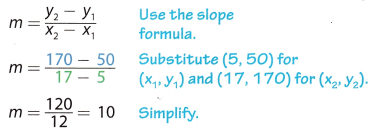STEP 2: Find the y-intercept of the trend line.STEP 3: Use your slope and y-intercept values to write the equation.
y = mx + b Slope-intercept form
y = 10x + 0 Substitute 10 for m and 0 for y.
The equation for the trend line is y = 10x.

Reflect

Question 3.
What type(s) of association does the scatter plot show?
The scatter plot shows a positive and linear association.

Question 4.
What is the meaning of the slope in this situation?
For every increase of two chapters, there is an increase of 20 pages.

Question 5.
What is the meaning of the y-intercept in this situation?
The y-intercept represents the total number of pages for several books. There are about 10 pages in a chapter.

Question 6.
The scatter plot and trend line show the relationship between the number of rainy days in a month and the number of umbrellas sold each month. Write an equation for the trend line.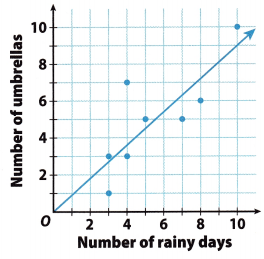The Line passes through (0, 0) and (10, 9).
Use the slope formula to find the slope
slope = $$\frac{y_{2}-y_{1}}{x_{2}-x_{1}}=\frac{9-0}{10-0}=\frac{9}{10}$$
The Line crosses the y-axis at (0, 0) so the y-intercept is 0
Form an equation for the trend line by substituting the value of the slope for m and the value of the y-intercept for b in the slope-intercept formula.
y = mx + b
y = $$\frac{9}{10}$$x + 0
y = $$\frac{9}{10}$$x

Explore Activity 2

Making Predictions

When you use a trend line or its equation to predict a value between data points that you already know, you interpolate the predicted value. When you make a prediction that is outside the data that you know, you extrapolate the predicted value.

Use the equation of the trend line in Example 1 to predict how many pages would be in a book with 26 chapters.
Is this prediction an example of interpolation or extrapolation? ____________
y = __________ Write the equation for your trend line.
y = __________ Substitute the number of chapters for x.
y = __________ Simplify.
I predict that a book with 26 chapters will have ____________ pages.

Reflect

Question 7.
Make a Prediction Predict how many pages would be in a book with 14 chapters. Is this prediction an example of interpolation or extrapolation?
Using the equation y = 10x, substitute 14 (chapters) for x to predict how many pages.
y = 10x = 10(14)
I predict there would be 140 pages.
Interpolation

Question 8.
Do you think that extrapolation or interpolation is more accurate? Explain.
Interpolation might be more accurate for linear data. With the given data, you can see the difference between points. If the surrounding points are close to the line, your prediction will be more accurate.

However, if the points have a large difference, interpolation may not give a good estimate.

Angela recorded the price of different weights of several bulk grains. She made a scatter plot of her data. Use the scatter plot for 1-4.Question 1.
Draw a trend line for the scatter plot. (Explore Activity 1)
Draw a trend line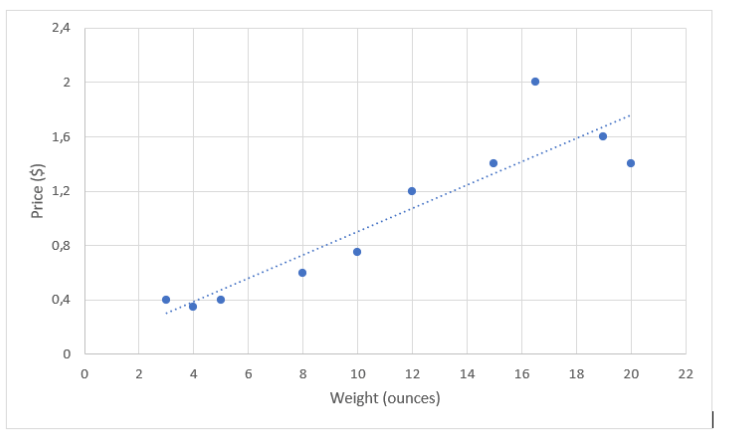Question 2.
How do you know whether your trend line is a good fit for the data? (Explore Activity 1)
Most of the data points are close to the trend line. The trend line has about the same number of points above and below it.

Question 3.
Write an equation for your trend line. (Example 1)
Step 1: The trend line passes through (0, 0) and (19, 1.80).

Step 2: Find the slope by using the slope formula.
slope = m = $$\frac{y_{2}-y_{1}}{x_{2}-x_{1}}=\frac{1.80-0}{19-0}=\frac{1.80}{19}$$ = 0.09

Step 3: The Line passes through the origin so the y-intercept is 0.

Step 4: Form an equation for the trend line by substituting the slope value for m and the value of the y-intercept for b in the stop-intercept formula.
y = mx + b
y = 0.09x + 0
y = 0.09x

Question 4.
Use the equation for your trend line to interpolate the price of 7 ounces and extrapolate the price of 50 ounces. (Explore Activity 2)
Use the equation for the trend Line (y = 0.09x) to interpolate the price of 7 ounces by substituting 7 for x (y = 0.09 ∙ 7) and solving for y.
Use the equation for the trend line (y = 0.09x) to extrapoLate the price of 50 ounces by substituting 50 for x (y = 0.09 ∙ 50)and solving for y.

Essential Question Check-In

Question 5.
A trend line passes through two points on a scatter plot. How can you use the trend line to make a prediction between or outside the given data points?
Use two points on the line. Find the slope and y-intercept. Substitute the vaLues of the slope (in) and y-intercept (b) to form an equation using y = mx + b. Substitute the value of x for which you want to make a prediction and solve for y OR substitute your prediction for y and solve for x to find its value.

Use the data in the table for Exercises 6-10.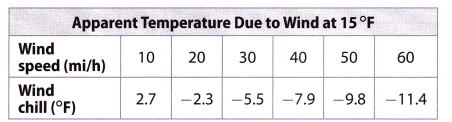Question 6.
Make a scatter plot of the data and draw a trend line.Make a scatter plot of the data and draw a trend line.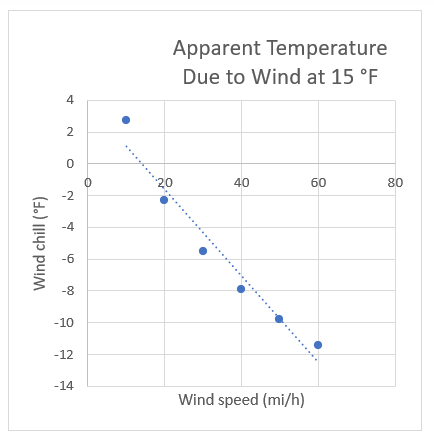Question 7.
What type of association does the trend line show?
One data set increases (Wind Speed) and the other (Wind Chill) decreases so the trend line shows a Negative Association.

Question 8.
Write an equation for your trend line.
Step 1:
m = $$\frac{y_{2}-y_{1}}{x_{2}-x_{1}}$$ Find the slope using the slope Formula.
= $$\frac{(-10)-(5)}{50-30}$$
= $$\frac{-5}{20}$$
= -0.25

Step 2:
y = mx + b Find the y-intercept using the Slope Intercept Formula.
-5 = -0.25(30) + b
-5 = -7.5 + b
2.5 = b

Step 3:
y = -0.25x + 2.5 Substitute the value of m and b into the Slope Intercept Formula to form an equation for the trend line.

Question 9.
Make a Prediction Use the trend line to predict the wind chill at these wind speeds.
a. 36 mi/h ___________
Use the trend line to predict the wind chill at 36 mi/h
y = -0.25x + 2.5
y = -0.25(36) + 2.5
y = – 9 + 2.5
y = -6.5
The wind chill at 36 mi/h is -6.5 °F.

b. 100 mi/h ____________
Use the trend line to predict the wind chill at 100 mi/h
y = -0.25x + 2.5
y = -0.25(100) + 2.5
y = -25 + 2.5
y = -22.5
The wind chill at 100 mi/h is 22.5 °F.

Question 10.
What is the meaning of the slope of the line?
The slope means that the wind chill falls about 1°F for every 4 mph increase in wind speed.

Use the data in the table for Exercises 11-14.Question 11.
Make a scatter plot of the data and draw a trend line.Make a scatter plot and draw a trend line.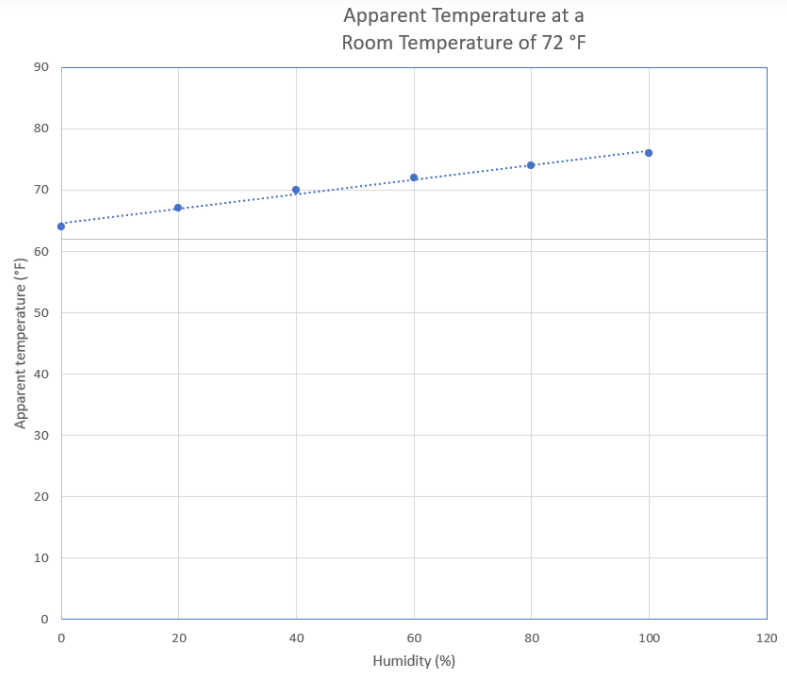Question 12.
Write an equation for your trend line.
Step 1:
Find the slope by using two points using the slope formula.
For example: (0, 64) and (60, 72).
m = $$\frac{y_{2}-y_{1}}{x_{2}-x_{1}}$$
= $$\frac{72-64}{60-0}$$
= $$\frac{8}{60}$$
= –$$\frac{2}{15}$$

Step 2:
The Line passes the y-axis at (0, 64).
The y-intercept = b = 64

Step 3:
Use the slope-intercept formuLa to form an equation for the trend line Substitute the slope value for m and the value of the y-intercept for b.
y = mx + b
y = –$$\frac{2}{15}$$x + 64

Question 13.
Make a Prediction Use the trend line to predict the apparent temperature at 70% humidity.
Use the equation of the trend line Substitute 70 (for 70%) into the equation for x.
y = –$$\frac{2}{15}$$x + 64
y = –$$\frac{2}{15}$$(70) + 64

Solve for y
y = –$$\frac{140}{5}$$ + 64
y ≈ –$$9 . \overline{3}$$ + 64
y ≈ $$73 . \overline{3}$$
The apparent temperature is 73.3° F.

Question 14.
What is the meaning of the y-intercept of the line?
The y-intercept means that at 0% humidity, the apparent temperature is 64°F.

H.O.T. Focus on Higher Order Thinking

Question 15.
Communicate Mathematical Ideas Is it possible to draw a trend line on a scatter plot that shows no association? Explain.
It is not possible to draw a trend line on a scatter plot that shows no association.

If the scatter plot shows no association, the data points have no relationships to one another. You can draw a trend line if there is a linear association.

Question 16.
Critique Reasoning Sam drew a trend line that had about the same number of data points above it as below it, but did not pass through any data points. He then picked two data points to write the equation for the line. Is this a correct way to write the equation? Explain.
Sam did not use the correct way to write an equation.
Sam may have drawn a correct trend line but using the data points that are not on the trend line may have an incorrect equation for the line. He should use two points on that trend line to write the equation.

Question 17.
Marlene wanted to find a relationship between the areas and populations • of counties in Texas. She plotted x (area in square miles) and y (population) for two counties on a scatter plot:
Kent County (903, 808)
Edwards County (2118, 2002)
She concluded that the population of Texas counties is approximately equal to their area in square miles and drew a trend line through her points.

a. Critique Reasoning Do you agree with Marlene’s method of creating : a scatter plot and a trend line? Explain why or why not.